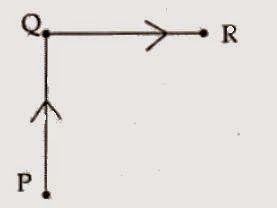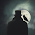SCIENCE - Class - IXTime Allowed : 3 hours -                                              Maximum Marks : 90

 General Instructions: 1. The question paper comprises of two Sections, A and B. You are to attempt both the sections. 2. All questions are compulsory 3. All questions of Section-A and all questions of Section-B are to be attempted separately. 4. Question numbers 1 to 3 in Section-A are one mark questions. These are to be answered in one word or in one sentence 5. Question numbers 4 to 6 in Sections-A are two marks questions. These are to be answered in about 30 words each. 6. Question numbers 7 to 18 in Section-A are three marks quesrons. These are to be answered in about 50 words each 7. Question numbers 19 to 24 in Section-A are five marks questions. These are to be answered in about 70 words each. 8. Question numbers 25 to 33 in Section-B are multiple choice questions based on practical skills. Each question is a one mark question. You are to select one most appropriate response out of the four provided to you. 9. Question numbers 34 to 36 in Section-B are questions based on practical skills are two marks questions.

 SECTION-A Q1. Which micro-organism lacks any kind of membrane ? 1 Q2. An ant travels from P to Q .and then moves from Q toR (as shown in the diagram). Show its resultant displacement in the diagram.1 Q3. Write the value of acceleration produced in a freely falling body. 1 Q4. State the principle used to separate two immiscible liquids of a mixture. Draw a neat and labelled diagram of the apparatus used. 2 Q5. What is the function of s\$xe tube cells and how are they designed to carry out their function ? 2 Q6. State reason for the following: (i) Road accidents occurring due to high speeds are much worse than accidents due to low speeds of vehicles. (ii) When a motor car makes a sharp turn at a high speed passengers tend to get thrown to one side. 2 Q7. (a) State one property of solid which liquid does not possess and one property of liquid which solid does not possess (b) List any two properties of gases. 3 Q8. Distinguish between a suspension and a colloidal solution in two ways a tabular form giving one example each. 3 Q9. Draw a well labeled diagram showing sublimation of Ammonium chloride. 3 Q10. How is a bacterial cell different from amoeba in terms of cellular arrangement? 3 Q11. (a) Differentiate between simple squamous epithelium and stratified squamous epithelium. (b) How epithelial tissue keeps different body systems separate? 3 Q12. The speed-time graph of a car is given. The car weighs 1000 kg. (a} What is the distance travelled by the car in first two seconds (b) What is the braking force applied at the end of 5 seconds to bring the car to a stop within one second. 3 Q13. Derive first law of motion mathematically from the mathematical expression for the second law of motion. 3 Q14. (i) State the condition under which the magnitude of average velocity of an object is equal to its average speed. (ii) Mention the type of motion exhibited by a free falling body. (iii)Explain giving one example a situation in which a body has a certain average speed buts its average velocity is zero. 3 Q15. A scooter starts from rest, moves in a straight line with a constant acceleration and covers a distance of 64 m in 4 s. (a) Calculate its acceleration and its final velocity. (b) At what time the scooter had covered half the total distance ? 3 Q16. If the distance between masses of two objects is increased by five units, by what factor would the mass of one of them have to be altered to maintain the same gravitational force? Would there be an increase or a decrease in the mass? 3 Q17. Ravi visited his village during vacation of school. He observed that elders of the village always talked about different cropping systems like mixed cropping, inter cropping and crop rotation. But they did not know the scientific reason behind these practices. Ravi explained them the scientific reason so that they could use them more gainfully. (i) What do we call this kind of farming system with minimal or no use of chemicals? (ii) Write the basis of selection of crops for inter cropping. (iii) Village elders appreciated Ravi. Give two possible reasons. 3 Q18. "There has been a 4 times increase in the production of food grains from 1960 to 2004 with only 25% increase in the cultivatable land area". Mention the steps undertaken by farmers to achieve this success. 3 Q19. (a) Answer the following:   (i) A sponge can be compressed yet it is a solid. Why ?   (ii) Out of honey or ink which will diffuse faster and why ? (b) List out three characteristics of particles of matter. 5 Q20. A student was given the mixture of Iron filings and sulphur. He was told to heat it and observe the following - (a) What is the colour of the compound formed? (b) Write the effect of magnet on it. (c) Write the action of carbon disulphide on it. (d) Describe the effect of adding dilute hydrochloric acid to it.Identify the gas and write its two properties 5 Q21. (a) Draw a neat diagram of an animal cell and label the parts whose functions are as follows:  (i)helps in storage modification and packaging of products in vesicles.  (ii) helps in keeping the cell clean by digesting work-out cell organelles.  (iii) helps in oxidation of glucose to produce energy in the form of ATP.  (iv) allows the entry and exit of some materials into and out of the cells. (b) Define nucleoid. 5 Q22. If the engine of a car provides an acceleration of 2 m/ s2 to start it from rest, assuming the mass to be roughly 1000 kg, calculate: (a) Force provided by the engine (b) Momentum after 10 sec. (c) Time after which the car comes to rest, if the engine is turned off after 15 sec. (frictional force=15 N) 5 Q23. (a) A motorcyclist riding motorcycle A is traveling at a speed of 20 m/s applies the brakes and stops the motorcycle in 10 s. Another motorcyclist of motorcycle B who is traveling at a speed of 5 m/s applies the brakes and stops the motorcycle in 20 s. Plot speed-time graph for the two motorcycles. Which of the two motorcycles travelled farther before it comes to a stop? (b) A cyclist goes around a circular track once every 2 minutes. If radius of the circular track is 110 m, calculate the speed of cyclist? 25 Q24. State the advantages of 'Inter Cropping' and 'Crop Rotation' over the technique of single crop. 5 SECTION -B Q25. The test tubes A, B, C and Dare taken with food samples of dal, mustard, soya crumbs and rice respectively in powdered form. On adding iodine solution to each test tube the blue black colour is observed in: (a) Test tube A (b) Test tube B (c) Test tube C (d) Test tube D 1 Q26. The presence of starch can be shown in which food material and using which reagent: (a)apple juice and Iodine solution (b) potato extract and safranin (c) apple juice and safranin potato extract and Iodine solution. 1 Q27. Which one of the following is an example of heterogeneous mixture ? (a) I (b) II (c) III (d) IV 1 Q28. On strongly heating a mixture of iron filings and sulphur powder a black mass is formed due to the formation of: (a) Hydrogen sulphide (b) Iron-oxide (c) Iron sulphide 1 Q29. When magnesium ribbon is burnt in air, the product formed mainly consists of: (a) magnesium nitrate (b) magnesium carbide (c) magnesium sulphide (d) magnesium oxide 1 Q30. While preparing a Temporary mount of an onion peel cells Sania mounted: (a) crushed pulp of onion (b) dry scale leaf (c) green leaf of onion (d) thin layer of fleshy leaf of onion. 1 Q31. The slide under a microscope shows alternate light and dark bands and many nuclei in the cells. It may most probably be of: (a) nerve cell (b)phloem fibres (c) striated muscle (d)sclerenchyma 1 Q32. Evaporation is the technique used to separate the components of a mixture of : (a) sodium chloride and glucose (b) sodium chloride and water (c) sodium chloride and ammonium chloride (d) glucose and ammonium chloride 1 Q33. The kind of force required to accelerate the motion of a body is : (a) Frictional force (b) Gravitational force (c) Unbalanced force (d) Balanced force. 1 Q34. Four students A, B, C and D were given funnels, filter paper, test tubes, test tube stands, common salt, chalk powder, starch and glucose powder. They prepared the true solution, suspension and colloidal solutions. Test tubes were arranged as shown in the figure. Observe the filtrate obtained in the test tubes and residue on filter paper. Conclude about filtrate, residue and type of solution. 1 Q35. Mention the type of thermometer that should be used to determine the melting point of ice in a laboratory. What should be the position of bulb of thermometer? 2 Q36. A student took x gram water in a beaker and dipped p gram of raisins in it. After keeping raisins in water for about 2 hours he measured the mass of soaked raisins as q grams. He also measured the mass of water left in the beaker which was y grams. On the basis of his observations write the correct formula to find the percentage of water absorbed by raisins. 21.very very very nice,helpful and intersing site

1.2.3.